# AdvDCSM System Tool and LOW-DENSITY PARITY-CHECK CODING

NOTE: This section is Under-Edit if necessary: Construction began on May 25, 2016 and was finished on June 16, 2016.

LOW-DENSITY PARITY-CHECK BINARY CODES: Signaling over a MLC & Iterative Message-Passing Channel Decoding

LOW-DENSITY PARITY-CHECK (LDPC) BINARY CODES: Signaling over a Memoryless Channel and Iterative Message-Passing Channel Decoding

LOW-DENSITY PARITY-CHECK BINARY CODES: Signaling over a Rayleigh Fading Memory Channel & Iterative Message-Passing Channel Decoding

LOW-DENSITY PARITY-CHECK (LDPC) BINARY CODES: Signaling over a Rayleigh Fading Memory Channel and the Iterative Message-Passing Channel Decoding

LOW-DENSITY PARITY-CHECK BINARY CODES: Signaling over a Parallel MultiChannel and Iterative Message-Passing Channel Decoding

LOW-DENSITY PARITY-CHECK (LDPC) BINARY CODES: Signaling over a Parallel MultiChannel and Iterative Message-Passing Channel Decoding

LOW-DENSITY PARITY-CHECK BINARY CODES: Irregular Gallager Coded Signaling over a Parallel MultiChannel and Iterative Message-Passing Channel Decoding

LOW-DENSITY PARITY-CHECK (LDPC) BINARY CODES: Irregular Gallager Coded Signaling over a Parallel MultiChannel and Iterative Message-Passing Channel Decoding

LOW-DENSITY PARITY-CHECK BINARY CODES: Irregular Gallager Coded (Column Weight Distribution) Signaling over a Parallel MultiChannel and Iterative Message-Passing Channel Decoding

LOW-DENSITY PARITY-CHECK (LDPC) BINARY CODES: Irregular Gallager Coded (Column Weight Distribution) Signaling over a Parallel MultiChannel and Iterative Message-Passing Channel Decoding

Low-Density Parity-Check (LDPC) Binary Codes: Signaling over a Memoryless Channel and Iterative Message-Passing Channel Decoding

```by Darrell A. Nolta
May 22, 2016 with June 16, 2016 Update
```

The AdvDCSMT1DCSS (T1) Professional (T1 Version 2) system tool now offers the capabilities to construct Gallager, Array, and Repeat-Accumulate (RA) Low-Density Parity-Check (LDPC) Binary Codes (Linear Block Codes) and to model and simulate Low-Density Parity-Check (LDPC) Coded Signaling over a Memoryless Channel (MLC) using these LDPC Codes. In addition to these capabilities, T1 V2 supports the capability of modeling and simulating Multiple Iteration Soft Input/Soft-Decision Output (SISO) LDPC Code Channel Decoding using the Sum-Product Algorithm (SPA). The SPA is a 'symbol-by-symbol' Maximum a Posteriori Probability (MAP) Belief Propagation Algorithm. Also, T1 V2 supports the capability to model and simulate the Multiple Iteration Hard Decision Bit Flipping Algorithm Decoder. These decoding algorithms can be used in the cases for simulated LDPC Coded Signaling over MLC with Additive White Gaussian Noise (AWGN) or LDPC Coded Signaling over BSC MLC.

In regards to the LDPC Codes construction capability, T1 Professional allows the User to construct certain Regular and Irregular Gallager Codes using the R.G. Gallager construction approach  & . T1 V2 creates these Gallager codes by using pseudorandom processes technique. T1 V2 implements a deterministic construction technique to compose certain structured LDPC codes: Array or truncated Array codes. Also, T1 V2 supports the construction of certain Regular and Irregular RA Codes by allowing the User to specify the Interleaver Permutation Mapping (Length <= 35) or using pseudorandom processes technique.

T1 V2 supports both LDPC Code Generator-based Encoding and Parity-Check Matrix H Encoding for Gallager and Array Coded Signaling.

The incorporation of the LDPC Coded M-ary Signaling over an AWGN MLC feature into T1 V2 supports Binary Modulation Schemes [Binary Phase Shift Keying (BPSK), Binary Pulse Amplitude Modulation (2-PAM), & Binary Frequency Shift Keying (BFSK)] and M > 2 Modulation Schemes (M-PSK, M-PAM, M-QAM, & M-FSK where M = 4, 8, 16, 32, and 64). M-QAM Signaling using a Square (M = 4, 16, or 64) or Non-Square (M = 8, 16, 32 or 64) Signal Vector Space can be used with a LDPC Code.

The SPA Decoder is based on: Model 1 (Bit Messages then Check Messages Iteration Processing) or Model 2 (Check Messages then Bit Messages Iteration Processing); Bit Estimation Likelihood Decision Rule: MAP (Maximum A Posteriori Probability) or ML (Maximum Likelihood) for an Unequiprobable Independent and Identical Distributed (i.i.d.) Binary Source; ML for Equiprobable i.i.d., All-Zero i.i.d., or Markov Binary Source; Implementation Type [for Check Message (Check Node to Bit Node Message) Calculation]: Theoretical SPA, Replace SPA Product Term by Sum, or Replace SPA Product Term by Min-Sum; and User Specified Maximum Number of SPA Decoding Iterations per Block (Imax): 3000 Maximum.

SPA LDPC Code Decoder's Figure-of-Merit consists of the Maximum Number of Iterations per Block (All Blocks) or Number of 'Detected Errors'; and Average (Sample Mean) and Sample Variance of Number of Iterations per Block.

Also, T1 V2 supports LDPC Coded M-ary Signaling Demodulation Constellation Demapping (M > 2 Search Algorithms): Search by Bit Position and Value Partition (Soft Decision) for LDPC Coded Single AWGN Memoryless Channels [Demodulation for LDPC Decoding Metric Calculation using Max-Log or Log-Sum Bit Metrics (User-specified)] & Constellation Demapper Decision Rule for LDPC Decoding: MAP or ML for an Unequiprobable i.i.d. Binary Source; ML for Equiprobable i.i.d., All-Zero i.i.d., or Markov Binary Source.

The Iterative Message-Passing SPA Channel Decoder implement a Stop Criterion that allows the Belief Propagation Decoder to stop iterating for each block of a set of transmitted code words (blocks) before the Maximum Number of Iterations per Block (Imax) is reached. The stopping event for the SPA Channel Decoder occurs when the Product of the Estimated Code Vector vest and Transpose of the Parity-Check Matrix H is equal to the Null Matrix 0.

The LDPC Encoding and Decoding algorithms development in T1 V2 was aided by the study of their description in a number of important works. Consult the References section at the end of this paper for some of these key works.

Consider the SPA Decoding Algorithm Bit Error Rate (BER) or Bit Error Probability Pb performance simulation results that are displayed below in Figures 1 through 11 plots. These results were produced by T1 V2 for LDPC Generator-based Encoding and Signaling. Figures 1, 2, 3, 4, 5, 6, 7, 8, and 11 plots correspond to Regular Gallager Coded (GC) BPSK Modulation. Figures 9 and 11 plots correspond to Array Coded (AYC) BPSK Modulation. Figures 10 and 11 plots correspond to Regular Repeat-Accumulate Coded (RAC) BPSK Modulation. Also, in addition to Pb results, Figure 1, 2, 3, and 7 displays the corresponding Average Number of Iterations per Block (Avg I). For comparison purposes, simulated BER results for UnCoded and Convolutional Coded (CC) (Rate = 1/2, 64-State) BPSK Signaling are included in each of these figures. Note that this Convolutional code is a NASA standard and provides an excellent reference in regards to its corresponding Viterbi Algorithm (VA) Channel Decoder complexity of 64 trellis states and its resulting BER performance.

Each figure's BER plot displays one or more curves where a curve is constructed from the set of simulated Pb values that correspond to a set of Eb/N0 [Signal-to-Noise Ratio (SNR)] values. Each GC, AYC, and RAC curve displays the performance behavior of a Regular Gallager, Array, and Regular Repeat-Accumulate Coding and Iterative Decoding system example that was used for a T1 V2 simulation.

Figure 1 displays the BER and Avg I versus Eb/N0 for different values of Imax (50 & 100) for (Code Vector Length, number of Code Bits (N) = 500, Column Weight (j) = 3, Row Weight (k) = 4) Gallager Coded Signaling for 10,000,107 Equal probable i.i.d. Information (Info) Bits.

Figure 2 displays the BER and Avg I versus Eb/N0 for different values of Imax (50 & 100) for (N = 500, j = 3, k = 5) Gallager Coded Signaling for 10,000,010 Equal probable i.i.d. Info Bits.

Figure 3 displays the BER and Avg I versus Eb/N0 for different values of Imax (50 & 100) for (N = 504, j = 3, k = 6) Gallager Coded Signaling for 10,000,234 Equal probable i.i.d. Info Bits.

Figure 4 displays the BER versus Eb/N0 for different values of Imax (50 & 100) for (N = 500, j = 3, k = 4) Gallager Coded Signaling for 10,000,107 and 1,000,125 Equal probable i.i.d. Info Bits.

Figure 5 displays BER versus Eb/N0 for different number of Imax (50 & 100) for (N = 500, j = 3, k = 5) Gallager Coded Signaling for 10,000,010 and 1,000,102 Equal probable i.i.d. Info Bits.

Figure 6 displays BER versus Eb/N0 for different number of Imax (50 & 100) for (N = 504, j = 3, k = 6) Gallager Coded Signaling for 10,000,234 and 1,000,252 Equal probable i.i.d. Info Bits.

Figure 7 displays BER versus Eb/N0 for different number of Imax (50 & 100) for (N = 504, j = 3, k = 6) Gallager Coded Signaling for 10,000,234 All-Zero i.i.d. Info Bits. The All-Zero Codeword is the only codeword transmitted.

Figure 8 displays BER versus Eb/N0 for different number of Imax (50 & 100) for (N = 504, j = 3, k = 6) Gallager Coded Signaling for 10,000,234 Equal probable i.i.d. Info Bits and 10,000,234 All-Zero i.i.d. Info Bits.

Figure 9 displays BER versus Eb/N0 for Imax of 50 for [N = 276, j = 3, k = 12, Identity & Permutation Matrices Dimension (prime number p) = 23, Permutation Matrix (alpha matrix) structure, a single cyclic shift version of Identity Matrix p x p: Right Cyclic Shift] and (N = 529, j = 3, k = 23, p = 23, Permutation Matrix: Right Cyclic Shift) Array Coded Signaling for 10,000,234 and 10,000,452 Equal probable i.i.d. Info Bits, respectively.

Figure 10 displays BER versus Eb/N0 for Imax of 50 for [N = 247, Source/Data Bits Vector Length, number of Source/Data Bits (K) = 208, Repetition code size (q) = 3, Combiner No. of Bits for Modulo 2 Sum (a) = 16] and (N = 247, K = 39, q = 16, a = 3) Repeat-Accumulate Coded Signaling for 10,000,016 and 10,000,029 Equal probable i.i.d. Info Bits, respectively.

Figure 11 displays BER versus Eb/N0 for Imax of 50 for (N = 529, j = 3, k = 23, p = 23, Permutation Matrix: Right Cyclic Shift) Array Coded Signaling, (N = 504, j = 3, k = 6) Gallager Coded Signaling, and (N = 247, K = 208) Repeat-Accumulate Coded Signaling for 10,000,452, 10,000,234, and 10,000,016 Equal probable i.i.d. Info Bits, respectively.

Note that for Gallager or Array Codes, L is synonymous with K, the number of Source/Data Bits per block or code vector.

There are a number of important conclusions that can be drawn from the below displayed simulated Iterative LDPC Code SPA Decoding BER results. It appears that T1 Professional is correctly modeling and simulating LDPC Coded Signaling over an AWGN Memoryless Channel with SPA Decoding for the selected Regular Gallager, Array, and Regular Repeat-Accumulate Codes.

One can clearly see the reduction in BER as the SNR is increased for the Gallager Coded BPSK Signaling with SPA Decoding (Imax = 50 & 100) as compared to the UnCoded BPSK Signaling with Maximum Likelihood Demodulation.

One can see from Figure 1,2, and 3 that only the (N = 504, j = 3, k = 6) Gallager Code with SPA Decoding outperforms the CC with VA Decoding.

From these BER results (Figure 4, 5, and 6), one can see the impact of the number of transmitted Information (Info) Bits on the accuracy (confidence of the BER estimate) of simulated BER results. Using 1 Million plus Info Bits, one could believe that for all three Gallager Codes (k = 4, 5, & 6) that they outperform the Rate = 1/2, 64-State Convolutional Code. But using 10 Million plus Info Bits, one obtains a different conclusion. Thus, to obtain accurate BER results for long block code lengths, simulation time could take many hours or days (depending on the User's computer). Note that Dr. Gallager discusses this issue in his 1962 paper  and 1963 book .

From these BER results (Figure 7 and 8), one can see the impact of the form of the transmitted codeword (Equal probable distribution of 'zeros' and 'ones' versus all 'zeros' makeup of a codeword). Figure 7 indicates that the (j = 3, k = 6, N = 504) Gallager code for the All-Zero Codeword transmission outperforms the Convolutional Code except for the region around Eb/N0 = 4.0 dB. Note that the BER value is zero for Eb/N0 equal to 4.3 dB and 4.5 dB.

A comparison of performance between the two forms of transmitted codewords is shown in Figure 8. Their BER curves track together fairly well except for the two SNR regions (~3 dB and ~4 dB). In these regions, the BER curves exhibit complex behavior (i.e., fluctuations for ~3 dB region & flat for ~4 dB region).

The above described T1 V2 simulated LDPC Coded Signaling BER results indicate that it is important (i.e., achieve reliable BER results) that a LDPC Code simulation use a large set of transmitted codewords where each codeword consists of a pseudorandom distribution of 'zeros' and 'ones'. The simulations of 10,000,234 Info Bits involved the transmission of 39,371codewords. But this is a very small number of codewords as compared to the possible set of codewords for a LDPC code. For example, consider the LDPC code of N = 504, L = 254, the number of possible codewords = 2254.

It is important to realize that R.G. Gallager used the All-Zero Codeword in obtaining his experimental results [2, Chapter 6]. He asserts that this approach is valid if the binary-input channel is symmetric and "that the actual simulation of decoding maintains complete symmetry between positive and negative outputs." In a practical LDPC Coded Signaling computer-based simulation, this complete symmetry cannot be achieved. This property is a function of the Gaussian pseudorandom number (RN) generator used in a simulation. The T1 V2's AWGN model characteristics for the 10,000,234 Info Bits simulation are as follows: Gaussian RN Mean = 0.000249, Gaussian RN Variance = 0.499955, Minimum Gaussian RN Value = -3.762706, and Maximum Gaussian RN Value = 3.902831.

But what is most interesting about these Gallager Code BER versus SNR plotted results is the observed BER fluctuations (i.e., the BER increases after the BER has declined with increasing SNR and then it declines as the SNR is further increased). One can see this behavior in Figure 2, 3, 5, 6 and 7, 8. Note that these fluctuations are very small relative to the number of Transmitted Information Bits (~1 Million or ~10 Million). And one observes that the Average Number of Iterations decrease montonically with increasing SNR with no fluctuations.

So what could be the possible cause of these BER fluctuations? Are they just random variations or some dependency of the decoding algorithm on the code parameters?

We must remember that the SPA decoder makes a decode decision on what most probable code word was transmitted given the channel output signal vector, source, and channel characteristics. This most probable codeword could be selected from (match) one of three possibilities: 1) the transmitted codeword, 2) a non-valid codeword (a 'detected error' codeword), or 3) a valid codeword other than the transmitted codeword (an 'undetected error' codeword).

It attempts to perform this decode decision using an iterating architecture that is based on the MxN Parity-Check Matrix that represents the set of M parity-check equations of a LDPC code. These equations define the BIT NODES, PARITY-CHECK NODES, and these nodes interrelationships (interconnections) architecture. Central to this function of the SPA decoder is that it attempts to solve a set of LDPC code parity-check equations in the process of generating the marginal posterior likelihoods for the code bits.

Key to the successful operation of a SPA Decoder is that its inputs [channel output signal (s) and extrinsic information signals] to BIT NODES are statistically independent (uncorrelated) to each other. This means that the corruption of each signal must not become correlated during the iterative process.

Now, the number of Info Bit Errors and the number of Detected Errors are indicators of SPA decoder performance for a given simulation SNR. The non-zero BER results are proof that the SPA decoder failed to decode some of the AWGN channel outputs. If the BER is non-zero and no Detected errors have occurred, the SPA decoder had converged to the wrong codeword (s) (undetected error events). If we look at the All-Zero codeword transmission cases, the experimental results inform us that undetected error (s) did occurs at SNR equal to 3.5, 4.0, 4.2, and 4.25 dB.

Let us focus on the nature of the SPA Decoder, i.e. its decoding algorithm is suboptimal globally even though the individual decoders (idealized model) are operating optimally. This fact means that there is not a unique solution to the LDPC Decoding problem, i.e., the composite decoder chooses an Estimated Codeword (a block of code bits) out of a set of possible Estimated Codewords. The Stopping Rule halts the decoding for a block when Convergence has occurred (i.e., the product of vest & HT is equal to the NULL Matrix, a bit-by-bit test). Convergence does not imply optimality of an estimated codeword (block) choice and its resulting code bits.

It is possible to have very complex convergence behavior occurring in the SPA decoding process that involves a small number of bit errors. Small error-prone substructures (EPSs) have been shown to exist in LDPC codes [13,15,16].

Next, one can see clearly in Figures 9 and 10 the reduction in BER as the SNR is increased for the Array Coded and Repeat-Accumulate Coded BPSK Signaling with SPA Decoding (Imax = 50) as compared to the UnCoded BPSK Signaling with ML Demodulation, respectively.

Let us examine the BER performance of the two Array Codes (N = 276 & N = 529) used in the T1 V2 evaluation of LDPC Coding and SPA Decoding that is display in Figure 9. One must note that these Array codes are High Rate codes, i.e., Rate = 0.757246 and 0.873346, respectively. First, both Array codes' performance is worst than the Rate = 1/2, 64-State CC performance. Second, the performance of the N = 276 Array code (Truncated) is better than the N = 529 Array code except at the SNR of 5.1 and 5.2 dB where their performance converges to be roughly identical. This behavior is complex given the different blocklengths and code rates. Note that each code's corresponding SPA Decoder uses the same number of Parity-Check Nodes since each code's Parity-Check Matrix H consists of the same number of rows (i.e., representing 69 parity-check equations). Each Parity-Check Node of the N = 276 code's SPA Decoder is connected to 12 Bit Nodes. For the N = 529 code, each SPA Decoder's parity-check node is connected to 23 Bit Nodes. In this case, the code's SPA Decoder with the smaller number of Bit Node to Parity-Check Node connections has better performance. The number of bits of redundancy for each code is 67. One might assert that the 67-bit redundancy of the N = 276 code provides more error correction capability than the 67-bit redundancy of the N = 529 code does.

In Figure 10, we examine the BER performance of two Regular Repeat-Accumulate (RA) codes with different code rates but have the same blocklength of 247 bits. The High Rate RA code (K = 208) has a Rate equal to 0.842105. The Low Rate RA code (K = 39) has a Rate equal to 0.157895. The High Rate RA code BER curve approximately matches the (N = 247, Rate = 0.84, random) RA code's BER curve (3.0 to 7.0 dB Eb/N0) in Figure 3 of the Johnson and Weller's 2005 paper . The performance for both RA codes is worst than the Rate = 1/2, 64-State CC performance. At SNR of 3.0 dB, the BER value for the Low Rate RA code is greater than the BER value for UnCoded BPSK. The performance for the High Rate RA code is better than the Low Rate RA code until the SNR reaches ~6.5 dB where their performance appears to converge together. For the SNR greater than ~6.5 dB, the performance of Low Rate code is better than the High Rate code. In regards to the number of bits of redundancy, the High Rate RA code has 39 bits and the Low Rate RA code has 208 bits. In this comparison, the Code Rate via the Demodulated Symbol SNR appears to have more effect on improving error correction capability than the number of bits of redundancy at the low end of SNR values.

Finally, in Figure 11, we compare the BER performance of the longest blocklength code for each LDPC code type used in this T1 V2 evaluation of LDPC Coding and SPA Decoding: (N = 504, Rate = 0.503968) Regular Gallager code, (N = 529, Rate = 0.873346) Array code, and (N = 247, Rate = 0.842105) Regular Repeat-Accumulate code. The Regular Gallager code outperforms the Rate = 1/2, 64-State CC as well as the Array and Regular Repeat-Accumulate codes. In regards to the number of bits of redundancy, the Regular Gallager code has 250 bits, the Array code has 67 bits, and the Regular Repeat-Accumulate code has 39 bits. The performance results for these three LDPC codes are consistent with the codes' number of bits of redundancy in terms of error correction capability as measured by the T1 Professional's simulated BER versus SNR parameters.

T1 Professional (T1 V2) now offers the LDPC Code (Gallager, Array, and Repeat-Accumulate) construction and LDPC Channel Coding and LDPC Channel Decoding (Iterative; based on the 'Symbol-by-Symbol' MAP Belief Propagation algorithm) for i.i.d. and Markov Information Binary Sources features to the User. The User via T1 V2 can get experience with the Generation of LDPC codes and the Sum-Product and Bit Flipping algorithms as applied to Iterative Decoding in simulated digital communication systems for Communications and Digital Storage Systems LDPC Coding applications.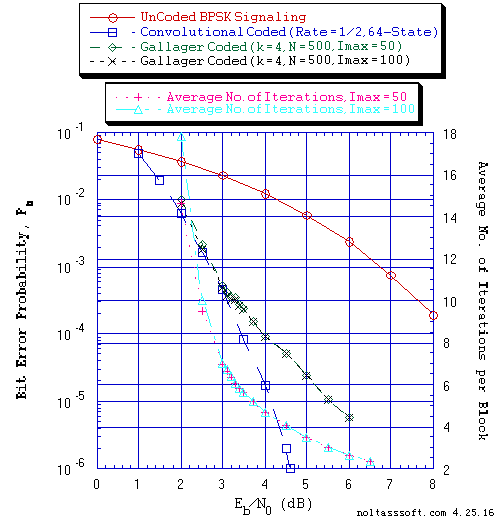```FIGURE 1. Bit Error Probability for UnCoded, Convolutional, and (N = 500, j = 3,
k = 4) Gallager Coded BPSK Signaling over a Coherent Memoryless Channel
with Additive White Gaussian Noise (AWGN):
```
```          Equal probable Independent and Identical Distributed Source for 10
Million, 1 Million, and 10,000,107 Information Bits for UnCoded,
Convolutional Coded, and Gallager Coded BPSK Signaling respectively
over a Vector Channel;
```
```          Rate = 1/2, Constraint Length K = 7 (3,1,0,3,3,2,3) a Best (Optimal)
Non-Recursive Convolutional Code (J.P. Odenwalder) and Viterbi Algorithm
using a Path Memory Length of 35 bits and an Unquantized Branch Metric;
```
```          N = 500, j = 3, k = 4, L = 127, Rate = 0.254 Regular Gallager Code
(T1 V2 Computer-generated); &
```
```          Sum-Product Algorithm Iterative Decoder using Model 2 (Check Messages
then Bit Messages Iteration Processing), Theoretical SPA Implementation
Type, and Maximum Number of Iterations per Block (Imax) = 50 & 100.
```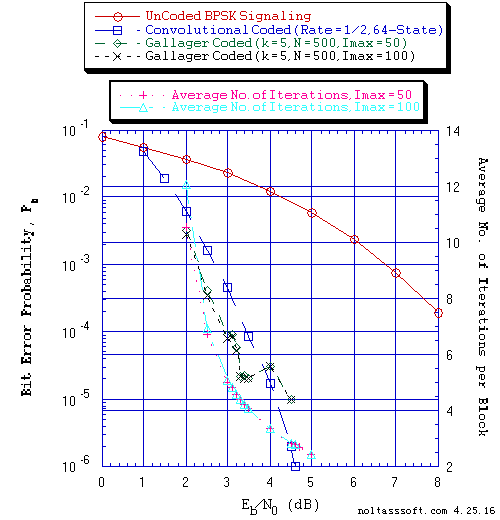```FIGURE 2. Bit Error Probability for UnCoded, Convolutional, and (N = 500, j = 3,
k =5) Gallager Coded BPSK Signaling over a Coherent Memoryless Channel
with Additive White Gaussian Noise (AWGN):
```
```          Equal probable Independent and Identical Distributed Source for 10
Million, 1 Million, and 10,000,010 Information Bits for UnCoded,
Convolutional Coded, and Gallager Coded BPSK Signaling respectively
over a Vector Channel;
```
```          Rate = 1/2, Constraint Length K = 7 (3,1,0,3,3,2,3) a Best (Optimal)
Non-Recursive Convolutional Code (J.P. Odenwalder) and Viterbi
Algorithm using a Path Memory Length of 35 bits and an Unquantized
Branch Metric;
```
```          N = 500, j = 3, k = 5, L = 202, Rate = 0.404 Regular Gallager Code
(T1 V2 Computer-generated); &
```
```          Sum-Product Algorithm Iterative Decoder using Model 2 (Check Messages
then Bit Messages Iteration Processing), Theoretical SPA Implementation
Type, and Maximum Number of Iterations per Block (Imax) = 50 & 100.
```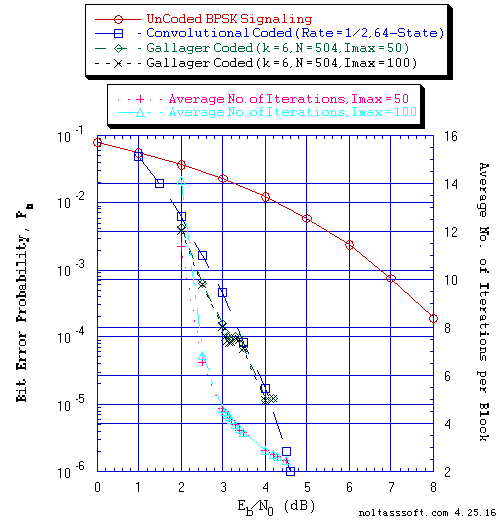```FIGURE 3. Bit Error Probability for UnCoded, Convolutional, and (N = 504, j = 3,
k = 6) Gallager Coded BPSK Signaling over a Coherent Memoryless Channel
with Additive White Gaussian Noise (AWGN):
```
```          Equal probable Independent and Identical Distributed Source for 10
Million, 1 Million, and 10,000,234 Information Bits for UnCoded,
Convolutional Coded, and Gallager Coded BPSK Signaling respectively
over a Vector Channel;
```
```          Rate = 1/2, Constraint Length K = 7 (3,1,0,3,3,2,3) a Best (Optimal)
Non-Recursive Convolutional Code (J.P. Odenwalder) and Viterbi Algorithm
using a Path Memory Length of 35 bits and an Unquantized Branch Metric;
```
```          N = 504, j = 3, k = 6, L = 254, Rate = 0.503968 Regular Gallager Code
(T1 V2 Computer-generated); &
```
```          Sum-Product Algorithm Iterative Decoder using Model 2 (Check Messages
then Bit Messages Iteration Processing), Theoretical SPA Implementation
Type, and Maximum Number of Iterations per Block (Imax) = 50 & 100.
```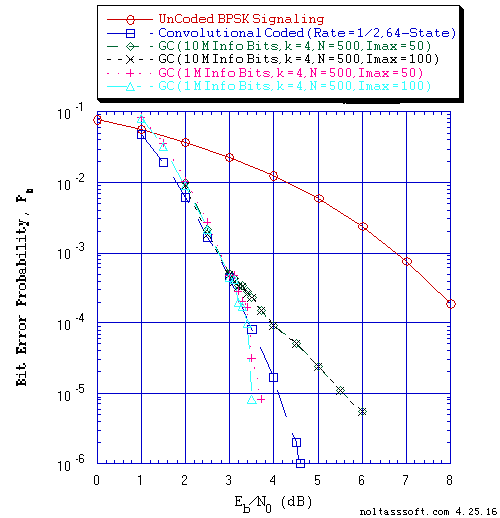```FIGURE 4. Bit Error Probability for UnCoded, Convolutional, and (N = 500, j = 3,
k = 4) Gallager Coded (GC) BPSK Signaling over a Coherent Memoryless
Channel with Additive White Gaussian Noise (AWGN):
```
```          Equal probable Independent and Identical Distributed (i.i.d.) Source
for 10 Million and 1 Million Information (Info) Bits for UnCoded and
Convolutional Coded BPSK Signaling respectively over a Vector Channel;
```
```          Equal probable i.i.d. Source for 10,000,107 and 1,000,125 Information
(Info) Bits for Gallager Coded BPSK Signaling respectively over a
Vector Channel;
```
```          Rate = 1/2, Constraint Length K = 7 (3,1,0,3,3,2,3) a Best (Optimal)
Non-Recursive Convolutional Code (J.P. Odenwalder) and Viterbi Algorithm
using a Path Memory Length of 35 bits and an Unquantized Branch Metric;
```
```          N = 500, j = 3, k = 4, L = 127, Rate = 0.254 Regular Gallager Code
(T1 V2 Computer-generated); &
```
```          Sum-Product Algorithm Iterative Decoder using Model 2 (Check Messages
then Bit Messages Iteration Processing), Theoretical SPA Implementation
Type, and Maximum Number of Iterations per Block (Imax) = 50 & 100.
```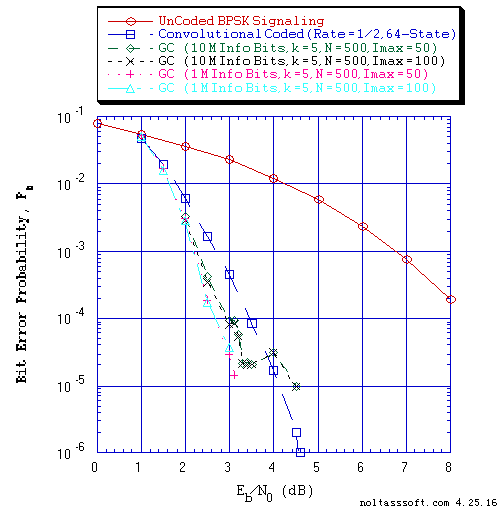```FIGURE 5. Bit Error Probability for UnCoded, Convolutional, and (N = 500, j = 3,
k = 5) Gallager Coded BPSK Signaling over a Coherent Memoryless
Channel with Additive White Gaussian Noise (AWGN):
```
```          Equal probable Independent and Identical Distributed  (i.i.d.) Source
for 10 Million and 1 Million Information (Info) Bits for UnCoded and
Convolutional Coded BPSK Signaling respectively over a Vector Channel;
```
```          Equal probable i.i.d. Source for 10,000,010 and 1,000,102 Information
(Info) Bits for Gallager Coded BPSK Signaling respectively over a
Vector Channel;
```
```          Rate = 1/2, Constraint Length K = 7 (3,1,0,3,3,2,3) a Best (Optimal)
Non-Recursive Convolutional Code (J.P. Odenwalder) and Viterbi Algorithm
using a Path Memory Length of 35 bits and an Unquantized Branch Metric;
```
```          N = 500, j = 3, k = 5, L = 202, Rate = 0.404 Regular Gallager Code
(T1 V2 Computer-generated); &
```
```          Sum-Product Algorithm Iterative Decoder using Model 2 (Check Messages
then Bit Messages Iteration Processing), Theoretical SPA Implementation
Type, and Maximum Number of Iterations per Block (Imax) = 50 & 100.
```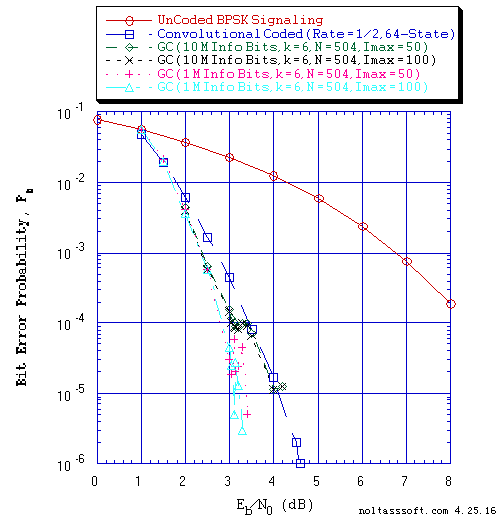```FIGURE 6. Bit Error Probability for UnCoded, Convolutional, and (N = 504, j = 3,
k = 6) Gallager Coded BPSK Signaling over a Coherent Memoryless
Channel with Additive White Gaussian Noise (AWGN):
```
```          Equal probable Independent and Identical Distributed  (i.i.d.) Source
for 10 Million and 1 Million Information (Info) Bits for UnCoded and
Convolutional Coded BPSK Signaling respectively over a Vector Channel;
```
```          Equal probable i.i.d. Source for 10,000,234 and 1,000,252 Information
(Info) Bits for Gallager Coded BPSK Signaling respectively over a
Vector Channel;
```
```          Rate = 1/2, Constraint Length K = 7 (3,1,0,3,3,2,3) a Best (Optimal)
Non-Recursive Convolutional Code (J.P. Odenwalder) and Viterbi Algorithm
using a Path Memory Length of 35 bits and an Unquantized Branch Metric;
```
```          N = 504, j = 3, k = 6, L = 254, Rate = 0.503968 Regular Gallager Code
(T1 V2 Computer-generated); &
```
```          Sum-Product Algorithm Iterative Decoder using Model 2 (Check Messages
then Bit Messages Iteration Processing), Theoretical SPA Implementation
Type, and Maximum Number of Iterations per Block (Imax) = 50 & 100.
```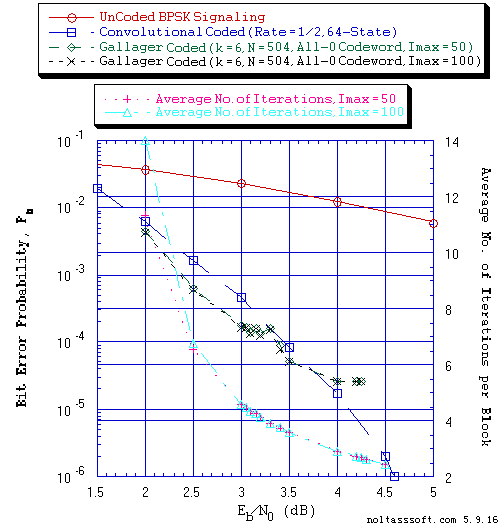```FIGURE 7. Bit Error Probability for UnCoded, Convolutional, and (N = 504, j = 3,
k = 6) Gallager Coded BPSK Signaling over a Coherent Memoryless
Channel with Additive White Gaussian Noise (AWGN):
```
```          Equal probable Independent and Identical Distributed (i.i.d.) Source
for 10 Million and 1 Million Information (Info) Bits for UnCoded and
Convolutional Coded BPSK Signaling respectively over a Vector Channel;
```
```          All-Zero i.i.d. Source for 10,000,234 Information (Info) Bits for
Gallager Coded (All-Zero Codeword) BPSK Signaling over a Vector Channel;
```
```          Rate = 1/2, Constraint Length K = 7 (3,1,0,3,3,2,3) a Best (Optimal)
Non-Recursive Convolutional Code (J.P. Odenwalder) and Viterbi Algorithm
using a Path Memory Length of 35 bits and an Unquantized Branch Metric;
```
```          N = 504, j = 3, k = 6, L = 254, Rate = 0.503968 Regular Gallager Code
(T1 V2 Computer-generated); &
```
```          Sum-Product Algorithm Iterative Decoder using Model 2 (Check Messages
then Bit Messages Iteration Processing), Theoretical SPA Implementation
Type, and Maximum Number of Iterations per Block (Imax) = 50 & 100.
```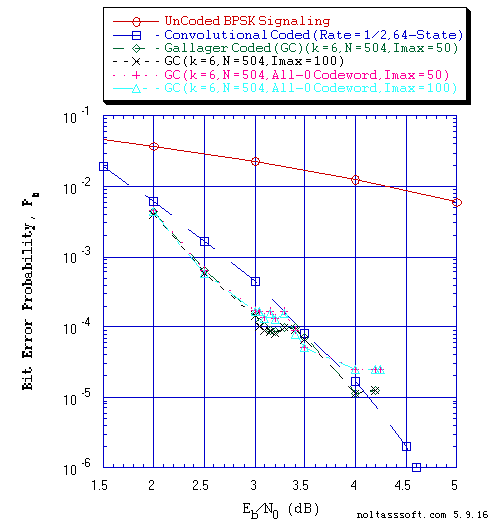```FIGURE 8. Bit Error Probability for UnCoded, Convolutional, and (N = 504, j = 3,
k = 6) Gallager Coded BPSK Signaling over a Coherent Memoryless
Channel with Additive White Gaussian Noise (AWGN):
```
```          Equal probable Independent and Identical Distributed (i.i.d.) Source
for 10 Million and 1 Million Information (Info) Bits for UnCoded and
Convolutional Coded BPSK Signaling respectively over a Vector Channel;
```
```          Equal probable i.i.d. Source for 10,000,234 Information (Info) Bits
for Gallager Coded BPSK Signaling over a Vector Channel;
```
```          All-Zero i.i.d. Source for 10,000,234 Information (Info) Bits for
Gallager Coded (All-Zero Codeword) BPSK Signaling over a Vector Channel;
```
```          Rate = 1/2, Constraint Length K = 7 (3,1,0,3,3,2,3) a Best (Optimal)
Non-Recursive Convolutional Code (J.P. Odenwalder) and Viterbi Algorithm
using a Path Memory Length of 35 bits and an Unquantized Branch Metric;
```
```          N = 504, j = 3, k = 6, L = 254, Rate = 0.503968 Regular Gallager Code
(T1 V2 Computer-generated); &
```
```          Sum-Product Algorithm Iterative Decoder using Model 2 (Check Messages
then Bit Messages Iteration Processing), Theoretical SPA Implementation
Type, and Maximum Number of Iterations per Block (Imax) = 50 & 100.
```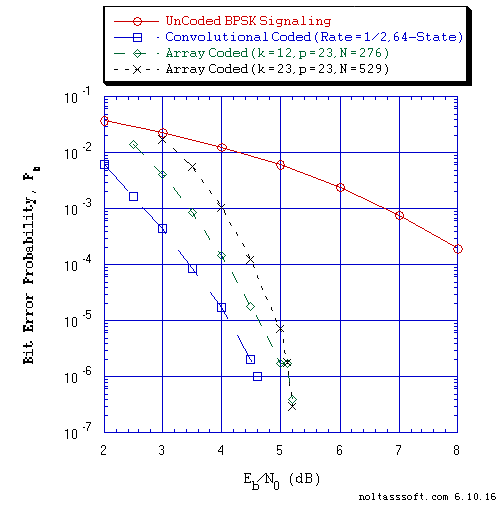```Figure 9. Bit Error Probability for UnCoded, Convolutional, and Array Coded BPSK
Signaling over a Coherent Memoryless Channel with Additive White Gaussian
Noise (AWGN):
```
```          Equal probable Independent and Identical Distributed (i.i.d.) Source
for 10 Million and 1 Million Information (Info) Bits for UnCoded and
Convolutional Coded BPSK Signaling respectively over a Vector Channel;
```
```          Equal probable i.i.d. Source for 10,000,023 and 10,000,452 Information
(Info) Bits for N = 276 and N = 529 Array Coded BPSK Signaling
respectively over a Vector Channel;
```
```          Rate = 1/2, Constraint Length K = 7 (3,1,0,3,3,2,3) a Best (Optimal)
Non-Recursive Convolutional Code (J.P. Odenwalder) and Viterbi
Algorithm using a Path Memory Length of 35 bits and an Unquantized
Branch Metric;
```
```          N = 276, j = 3, k = 12, p = 23, Right Cyclic Shift alpha matrix,
L = 209, Rate = 0.757246 Array Code (T1 V2 Computer-generated);
```
```          N = 529, j = 3, k = 23, p = 23, Right Cyclic Shift alpha matrix,
L = 462, Rate = 0.873346 Array Code (T1 V2 Computer-generated); &
```
```          Sum-Product Algorithm Iterative Decoder using Model 2 (Check Messages
then Bit Messages Iteration Processing), Theoretical SPA Implementation
Type, and Maximum Number of Iterations per Block (Imax) = 50.
```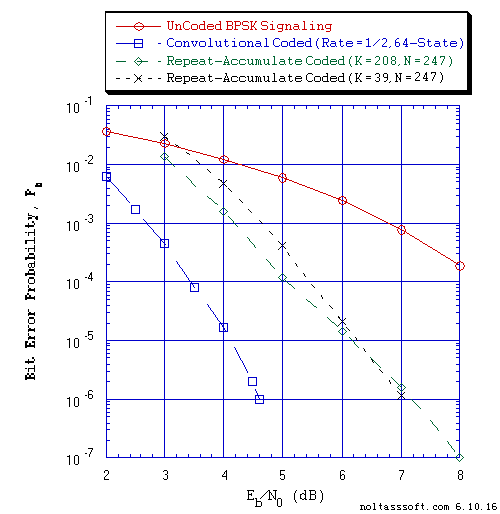```Figure 10. Bit Error Probability for UnCoded, Convolutional, and Regular
Repeat-Accumulate Coded BPSK Signaling over a Coherent Memoryless
Channel with Additive White Gaussian Noise (AWGN):
```
```           Equal probable Independent and Identical Distributed (i.i.d.) Source
for 10 Million and 1 Million Information (Info) Bits for UnCoded and
Convolutional Coded BPSK Signaling respectively over a Vector Channel;
```
```           Equal probable i.i.d. Source for 10,000,029 and 10,000,016 Information
(Info) Bits for N = 247, K = 208 and N = 247, K = 39 Regular
Repeat-Accumulate Coded BPSK Signaling respectively over a Vector Channel;
```
```           Rate = 1/2, Constraint Length K = 7 (3,1,0,3,3,2,3) a Best (Optimal)
Non-Recursive Convolutional Code (J.P. Odenwalder) and Viterbi
Algorithm using a Path Memory Length of 35 bits and an Unquantized
Branch Metric;
```
```           N = 247, K = 208, q = 3, a = 16, Rate = 0.842105 Regular Repeat-Accumulate
Code (T1 V2 Computer-generated);
```
```           N = 247, K = 39, q = 16, a = 3, Rate = 0.157895 Regular Repeat-Accumulate
Code (T1 V2 Computer-generated); &
```
```           Sum-Product Algorithm Iterative Decoder using Model 2 (Check Messages
then Bit Messages Iteration Processing), Theoretical SPA Implementation
Type, and Maximum Number of Iterations per Block (Imax) = 50.
```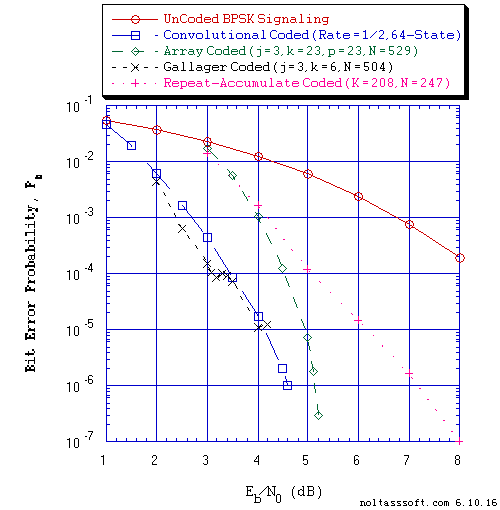```Figure 11. Bit Error Probability for UnCoded, Convolutional, and Low-Density
Parity-Check Coded BPSK Signaling over a Coherent Memoryless Channel
with Additive White Gaussian Noise (AWGN):
```
```           Equal probable Independent and Identical Distributed (i.i.d.) Source
for 10 Million and 1 Million Information (Info) Bits for UnCoded and
Convolutional Coded BPSK Signaling respectively over a Vector Channel;
```
```           Equal probable i.i.d. Source for 10,000,452, 10,000,234, and 10,000,016
Information (Info) Bits for N = 529 Array, N = 504 Regular Gallager,
and N = 247 Repeat-Accumulate Coded BPSK Signaling respectively over a
Vector Channel;
```
```           Rate = 1/2, Constraint Length K = 7 (3,1,0,3,3,2,3) a Best (Optimal)
Non-Recursive Convolutional Code (J.P. Odenwalder) and Viterbi
Algorithm using a Path Memory Length of 35 bits and an Unquantized
Branch Metric;
```
```           N = 529, j = 3, k = 23, p = 23, Right Shift, L = 462, Rate = 0.873346
Array Code (T1 V2 Computer-generated);
```
```           N = 504, j = 3, k = 6, L = 254, Rate = 0.503968 Regular Gallager Code
(T1 V2 Computer-generated);
```
```           N = 247, K = 208, q = 3, a = 16, Rate = 0.842105 Regular Repeat-Accumulate
Code (T1 V2 Computer-generated); &
```
```           Sum-Product Algorithm Iterative Decoder using Model 2 (Check Messages
then Bit Messages Iteration Processing), Theoretical SPA Implementation
Type, and Maximum Number of Iterations per Block (Imax) = 50.
```
References:

 Robert G. Gallager, "Low-Density Parity-Check Codes," IRE Transactions on Information Theory, Vol. IT-8, pp. 21-28, January 1962.

 Robert G. Gallager, Low-Density Parity-Check Codes, Number 21 of the M.I.T. Press Research Monographs, M.I.T. Press, Cambridge, Massachusetts, 1963.

 D.J.C. MacKay and R.M. Neal, "Near Shannon limit performance of low density parity check codes," Electronics Letters, Vol. 32, No. 18, pp. 1645-1646, August 29 1996.

 Matthew C. Davey and David J.C. MacKay, "Low Density Parity Check Codes over GF(q)," IEEE Communications Letters, Vol. 2, Issue: 6, pp. 165-167, June 1998.

 D.J.C. MacKay, "Good error-correcting codes based on very sparse matrices," IEEE Transactions on Information Theory, Vol. 45, No. 2, pp. 399 - 431, March 1999.

 John L. Fan, Constrained Coding and Soft Iterative Decoding, Kluwer Academic Publishers, Boston, 2001.

 E. Eleftherious and S. Olcer,"G.gen: LDPC codes for G.dmt.bis and G.lite.bis", http://web.stanford.edu/class/ee379b/class_reader/ibm3.pdf, January 2001.

 Thomas J. Richardson and Rudiger L. Urbanke, "Efficient Encoding of Low-Density Parity-Check Codes," IEEE Transactions on Information Theory, Vol. 47, No. 2, pp. 638 - 656, February 2001.

 Yu Kou, Shu Lin, and Marc P.C. Fossorier, "Low-Density Parity-Check Codes Based on Finite Geometries: A Rediscovery and New Results," IEEE Transactions on Information Theory, Vol. 47, No. 7, pp. 2711-2736, November 2001.

 Shu Lin and Daniel J. Costello, Jr., Error Control Coding: fundamentals and applications, Second Edition, Pearson Prentice Hall, Upper Saddle River, New Jersey, 2004.

 Sarah J. Johnson and Steven Weller, "Interleaver and Accumulator Design for Systematic Repeat-Accumulate Codes," 2005 Australian Communications Theory Workshop (Brisbane, Qld.), pp. 1-7, Feb 2-4, 2005.

 Sarah J. Johnson and Steven Weller, "Constructions for Irregular Repeat-Accumulate Codes", in Proc., International Symposium on Information Theory (Adelaide,SA), pp. 179-183, September 4-9 2005.

 Chih-Chun Wang, Sanjeev R. Kulkarni, and H. Vincent Poor, "Finding All Small Error-Prone Substructures in LDPC Codes," IEEE Transactions on Information Theory, Vol. 55, No. 5, pp. 1976-1998, May 2009.

 Sarah J. Johnson, Iterative Error Correction Turbo, Low-Density Parity-Check and Repeat-Accumulate Codes, Cambridge University Press, New York, 2010.

 Xiaojie Zhang and Paul H. Siegel, "Efficient Algorithms to Find All Small Error-Prone Substructures in LDPC Codes," in Proc., IEEE Globecom Conference (Houston, TX), pp. 1-6, December 2011.

 Gyu Bum Kyung and Chih-Chun Wang, "Finding the Exhaustive List of Small Fully Absorbing Sets and Desigining the Corresponding Low Error-Floor Decoder," IEEE Transactions on Communications, Vol. 60, No. 6, pp. 1487-1498, June 2012.

```BUY T1 Version 2 (ADVDCSMT1DCSS Professional software system tool)NOW.
```